Search by Topic

Resources tagged with Mathematical modelling similar to Procedure Solver:

Filter by: Content type:
Age range:
Challenge level:

There are 69 results

Broad Topics > Using, Applying and Reasoning about Mathematics > Mathematical modellingAn Introduction to Computer Programming and Mathematics

Age 16 to 18

This article explains the concepts involved in scientific mathematical computing. It will be very useful and interesting to anyone interested in computer programming or mathematics.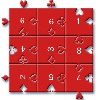Impuzzable

Age 16 to 18

This is about a fiendishly difficult jigsaw and how to solve it using a computer program.Shaping the Universe III - to Infinity and Beyond

Age 11 to 16

The third installment in our series on the shape of astronomical systems, this article explores galaxies and the universe beyond our solar system.Snooker

Age 16 to 18 Challenge Level:

A player has probability 0.4 of winning a single game. What is his probability of winning a 'best of 15 games' tournament?In Constantly Passing

Age 14 to 16 Challenge Level:

A car is travelling along a dual carriageway at constant speed. Every 3 minutes a bus passes going in the opposite direction, while every 6 minutes a bus passes the car travelling in the same. . . .Bionrich

Age 14 to 18 Challenge Level:

bioNRICH is the area of the stemNRICH site devoted to the mathematics underlying the study of the biological sciences, designed to help develop the mathematics required to get the most from your. . . .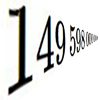Big and Small Numbers in Physics - Group Task

Age 16 to 18 Challenge Level:

Work in groups to try to create the best approximations to these physical quantities.Engnrich

Age 16 to 18 Challenge Level:

engNRICH is the area of the stemNRICH Advanced site devoted to the mathematics underlying the study of engineeringPhysnrich

Age 14 to 18 Challenge Level:

PhysNRICH is the area of the StemNRICH site devoted to the mathematics underlying the study of physicsRamping it Up

Age 16 to 18 Challenge Level:

Look at the calculus behind the simple act of a car going over a step.Chemnrich

Age 14 to 18 Challenge Level:

chemNRICH is the area of the stemNRICH site devoted to the mathematics underlying the study of chemistry, designed to help develop the mathematics required to get the most from your study. . . .Rocking Chairs, Railway Games and Rayboxes

Age 5 to 18

In this article for teachers, Alan Parr looks at ways that mathematics teaching and learning can start from the useful and interesting things can we do with the subject, including. . . .Age 16 to 18 Challenge Level:

This is the section of stemNRICH devoted to the advanced applied mathematics underlying the study of the sciences at higher levelsPredator - Prey Systems

Age 16 to 18 Challenge Level:

See how differential equations might be used to make a realistic model of a system containing predators and their prey.Modelling Assumptions in Mechanics

Age 16 to 18

An article demonstrating mathematically how various physical modelling assumptions affect the solution to the seemingly simple problem of the projectile.The Wrong Stats

Age 16 to 18 Challenge Level:

Why MUST these statistical statements probably be at least a little bit wrong?The Not-so-simple Pendulum 1

Age 16 to 18 Challenge Level:

See how the motion of the simple pendulum is not-so-simple after all.Twenty20

Age 7 to 16 Challenge Level:

Fancy a game of cricket? Here is a mathematical version you can play indoors without breaking any windows.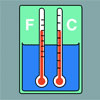Stemnrich - the Physical World

Age 11 to 16 Challenge Level:

PhysNRICH is the area of the StemNRICH site devoted to the mathematics underlying the study of physicsStringing it Out

Age 14 to 16 Challenge Level:

Explore the transformations and comment on what you find.Pdf Stories

Age 16 to 18 Challenge Level:

Invent scenarios which would give rise to these probability density functions.Population Dynamics

Age 16 to 18 Challenge Level:

This problem opens a major sequence of activities on the mathematics of population dynamics for advanced students.Branching Processes and Extinction

Age 16 to 18 Challenge Level:

An advanced mathematical exploration supporting our series of articles on population dynamics for advanced students.Population Dynamics - Part 1

Age 16 to 18 Challenge Level:

First in our series of problems on population dynamics for advanced students.Population Dynamics - Part 2

Age 16 to 18 Challenge Level:

Second in our series of problems on population dynamics for advanced students.Population Dynamics - Part 3

Age 16 to 18 Challenge Level:

Third in our series of problems on population dynamics for advanced students.Population Dynamics - Part 4

Age 16 to 18 Challenge Level:

Fourth in our series of problems on population dynamics for advanced students.Population Dynamics - Part 5

Age 16 to 18 Challenge Level:

Fifth in our series of problems on population dynamics for advanced students.Population Dynamics - Part 6

Age 16 to 18 Challenge Level:

Sixth in our series of problems on population dynamics for advanced students.Population Ecology Using Probability

Age 16 to 18 Challenge Level:

An advanced mathematical exploration supporting our series of articles on population dynamics for advanced students.Population Dynamics Collection

Age 16 to 18 Challenge Level:

This is our collection of tasks on the mathematical theme of 'Population Dynamics' for advanced students and those interested in mathematical modelling.Guessing the Graph

Age 14 to 16 Challenge Level:

Can you suggest a curve to fit some experimental data? Can you work out where the data might have come from?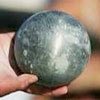Model Solutions

Age 16 to 18 Challenge Level:

How do these modelling assumption affect the solutions?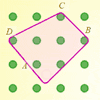Elastic Maths

Age 14 to 18

How do you write a computer program that creates the illusion of stretching elastic bands between pegs of a Geoboard? The answer contains some surprising mathematics.The Legacy

Age 14 to 16 Challenge Level:

Your school has been left a million pounds in the will of an ex- pupil. What model of investment and spending would you use in order to ensure the best return on the money?The Mean Game

Age 16 to 18

Edward Wallace based his A Level Statistics Project on The Mean Game. Each picks 2 numbers. The winner is the player who picks a number closest to the mean of all the numbers picked.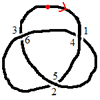Drawing Doodles and Naming Knots

Age 7 to 18

This article for students introduces the idea of naming knots using numbers. You'll need some paper and something to write with handy!What's a Knot?

Age 7 to 16 Challenge Level:

A brief video explaining the idea of a mathematical knot.Cushion Ball

Age 16 to 18 Challenge Level:

The shortest path between any two points on a snooker table is the straight line between them but what if the ball must bounce off one wall, or 2 walls, or 3 walls?Stonehenge

Age 16 to 18 Challenge Level:

Explain why, when moving heavy objects on rollers, the object moves twice as fast as the rollers. Try a similar experiment yourself.Fixing the Odds

Age 14 to 16 Challenge Level:

You have two bags, four red balls and four white balls. You must put all the balls in the bags although you are allowed to have one bag empty. How should you distribute the balls between the two. . . .Escalator

Age 14 to 16 Challenge Level:

At Holborn underground station there is a very long escalator. Two people are in a hurry and so climb the escalator as it is moving upwards, thus adding their speed to that of the moving steps. . . .
Concrete Calculation

Age 14 to 16 Challenge Level:

The builders have dug a hole in the ground to be filled with concrete for the foundations of our garage. How many cubic metres of ready-mix concrete should the builders order to fill this hole to. . . .Over-booking

Age 16 to 18 Challenge Level:

The probability that a passenger books a flight and does not turn up is 0.05. For an aeroplane with 400 seats how many tickets can be sold so that only 1% of flights are over-booked?Scratch Cards

Age 14 to 16 Challenge Level:

To win on a scratch card you have to uncover three numbers that add up to more than fifteen. What is the probability of winning a prize?Overarch 2

Age 16 to 18 Challenge Level:

Bricks are 20cm long and 10cm high. How high could an arch be built without mortar on a flat horizontal surface, to overhang by 1 metre? How big an overhang is it possible to make like this?Chocolate 2010

Age 14 to 16 Challenge Level:

First of all, pick the number of times a week that you would like to eat chocolate. Multiply this number by 2...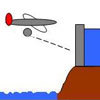Dam Busters 1

Age 16 to 18 Challenge Level:

At what positions and speeds can the bomb be dropped to destroy the dam?Shaping the Universe I - Planet Earth

Age 11 to 16

This article explores ths history of theories about the shape of our planet. It is the first in a series of articles looking at the significance of geometric shapes in the history of astronomy.Spot the Card

Age 14 to 16 Challenge Level:

It is possible to identify a particular card out of a pack of 15 with the use of some mathematical reasoning. What is this reasoning and can it be applied to other numbers of cards?